Students can access the CBSE Sample Papers for Class 12 Chemistry with Solutions and marking scheme Term 2 Set 3 will help students in understanding the difficulty level of the exam.

## CBSE Sample Papers for Class 12 Chemistry Term 2 Set 3 with Solutions

Time Allowed: 2 Hours
Maximum Marks: 35

General Instructions:

• There are 12 questions in this question paper with internal choice.
• SECTION A – Q. No. 1 to 3 are very short answer questions carrying 2 marks each.
• SECTION B – Q. No. 4 to 11 are short answer questions carrying 3 marks each.
• SECTION C- Q. No. 12 is case based question carrying 5 marks.
• All questions are compulsory.
• Use of log tables and calculators is not allowed.

Section – A
(Section A-Question No 1 to 3 are very short answer questions carrying 2 marks each.)

Question 1.
(A) Devise the correct cell representation for the given cell reaction?
Zn + H2SO4 → ZnS04 + H2
The Correct representation of the cell is , Zn|Zn2+ || H+, H2 | Pt

Caution:
In cell representation oxidation is represented in left part and the reduction in night. It has to be remembered that H2 electrode has Pt too in it.

(B) Why is it not possible to measure single electrode potential? (2)
It is not possible to measure single electrode potential because the half cell containing a single electrode cannot exist independently, as the charge cannot flow on its own in a single electrode.

Question 2.
Answer the following questions- (any two)
(A) You see a small packet inserted in a vitamin bottles or leather products, what is its role?
Little packets of silica gel are found in all sorts of products because silica gel is a desiccant it adsorbs and holds water vapour. In leather products and foods like pepperoni, the lack of moisture can limit the growth of mold and reduce spoilage, If a bottle of vitamins contained any moisture and were cooled rapidly, the condensing moisture would ruin the pills hence silica gel is added to adsorb moisture.

(B) How is a delta created?
Rivers are negatively charged colloidal solutions of silicates dispersed in water and sea water contain positively charged calcium and magnesium ions. When the two meet coagulation occurs and a delta is formed by deposition of particles.

Related Theory:
Coagulation it is a process of aggregating together the colloidal particle so as to change them into large sized particles which ultimately setties as precipitate. The coagulation is generally brought about by the addition of electrolytes.

(C) Critical temperatures of N2, SO2 and CH4 are 126, 230,110K respectively. Arrange them in increasing order of adsorption on the surface of activated charcoal. (2)
Extent of adsorption is directly proportional to critical temperature. Hence the order of extent of adsorption of the given gases is: SO2 > CH4 > N2

Related Theory:
The critical temperature of a substance can be defined as the highest temperature at which the substance can exist as a liquid. At temperatures above the critical temperature, the substance in question (in its vapour/gaseous state) can no longer be liqufied, regardless of the amountt pressure applied to it.Question 3.
(A) Give the product for: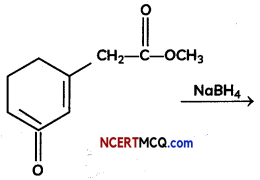NaBH4 does not reduce ester group. It is mild reducing reagent which reduces aldehyde and ketone into their respective alcohols.(B) Which among the following will undergo connizaro reciction?
Benzaldehyde, Acetone, Formaldehyde (2)
Benzaldehyde and formaldehyde undergo cannizaro reaction as these compounds do not contain alpha hydrogen

Related Theory:
An alpha carbon is the first carbon that is joined to the functional group, in the case of aldehydes and ketones, a functional group is a carbonyl group. The functional group is a carbonyl group, the functional group is responsible for the formation of alpha hydrogen.Section – B
(Section B-Question No 4 to 11 are short answer questions carrying 3 marks each.)

Question 4.
The electrical resistance of a column of 0.05 M NaOH solution of diameter 1 cm and length 50 cm is 5.55 × 103 ohm. Calculate its resistivity, conductivity and molar conductivity. (3)
A = πr2 = 3.14 × (0.5)2 cm2 = 0.785 cm2 = 0.785 × 10-4 m2
l = 50 cm = 0.5 mIf we want to calculate the values of different quantities in terms of ‘m’ instead of ‘cm’.Question 5.
The following graphs represents concentration of reactants versus time for a first-order reaction.
(A) What is the value of slope and intercept?
(B) Calculate the rate constant if the half-life of a first-order reaction was found to be 10 min at a certain temperature.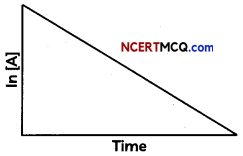OR
Show that time required for 99% completion is twice the time required for the completion of 90% reaction. (3)
(A) In [A] – In [A]0 = -kt
Rearrange to solve for [A] to obtain one form of the rate law; ln[A]0 = ln[A]0 – kt

This can further be arranged into y = mx +b form;
ln [A] = -kt + ln[A]0

The equation is a straight line with slope m will be equal: to negative of rate constant mx = -kt so slope = -k and y-intercept b will be equal to b= ln[A]0

(B) For first order reaction
k = $$\frac{0.693}{t_{1 / 2}}$$, where t1/2 = 600 s = 10 min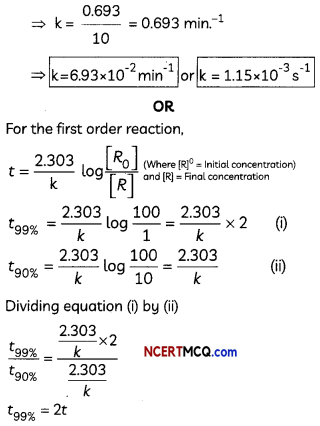Question 6.
Observe the experimental set up below and answer the following questions: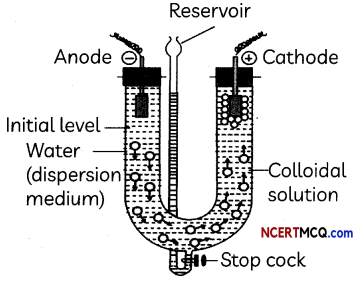(A) What happens when an electric current is passed through a colloidal solution?
On passing electric current through a sol, colloidal particles start moving towards oppositely charged electrode where they lose their charge and get coagulated (electrophoresis).

(B) What will happen if this movement of particles is prevented?
When the movement of particles is prevented, it is observed that the dispersion medium starts to move in the electric field. This is called electroosmosis.

Related Theory
Electrophoresis, which is one of the electrokinetic phenomena observed in colloidal systems, is the motion of charged colloidal particles in a liquid medium under an applied electric field.

(C) Name the process and write its one application. (3)
Process is electrophoressis by which lyophobic colloids can be coagulated.

Question 7.
(A) What is Tollen’s reagent? Write one usefulness of this reagent.
(B) An organic compound A has the molecular folmula C8H16O2. It gets hydrolysed with dilute sulphuric acid and gives a carboxylic acid B and an alcohol C. Oxidation of C with chromic acid also produced B. C on dehydration reaction gives but-l-ene. Write equations for the reactions involved. (3)
Ammonical silver nitrate solution is called Tollen’s reagent. [Ag (NH3)2]OH Uses: It is used to test aldehydes. Both aliphatic and aromatic aldehydes reduce Tollen’s reagent to shining silver mirror. It is also used to distinguish aldehydes from ketones.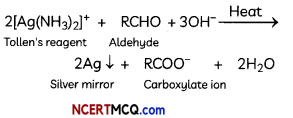(B) The compound A with molecular formula is Butyl butanoate.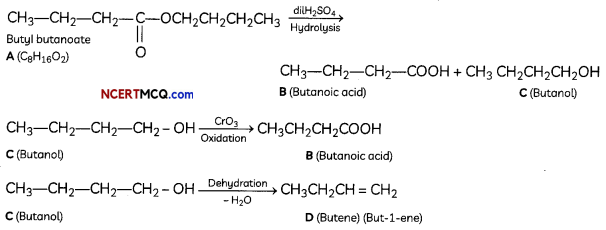Question 8.Compare the following complexes with respect to structural shapes of units, magnetic behaviour and hybrid orbitals involved in units:
[CO(NH3)6]+3, [Cr(NH3)6]+3, Ni(CO)4 (At. nos.: Co = 27, Cr = 24, Ni = 28
OR
What is meant by crystal field splitting energy? On the basis of crystal field theory, write the electronic configuration of d4 terms of t2g and eg in an octahedral field when (3)
(i) ∆0 > p
(ii) ∆0 < p
(i) [Co(NH3)6]+3 → Octahedral shape, d2sp3 hybridisation, diamagnetic
Formation of [Co(NH3)6]+3 → oxidation state of Co is +3.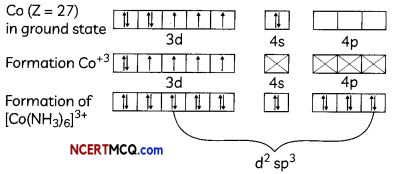(ii) [Cr(NH3)6]+3
Octahedral shape, d2sp3 hybridisation poramagnetic (3 unpaired electrons)(iii) [Ni(CO)4]
Tetrahedral shape, sp3 hybridisation. diamagnetic (no unpaired eLectrons)Or

Crystal field splitting energy: When ligands approach the central metal ion, the degenerate d-orbitals split into two sets, one with lower energy (t2g) and the other with higher energy (eg). The difference of energy between these two sets of orbitals is called crystal field splitting energy. (∆0 for octahedral complexes). The magnitude of ∆0 decides the actual configuration of d-orbitals by the help of mean pairing energy.

(i) If P > ∆0 then pairing of electrons does not occur and electrons enter in the higher energy eg orbitals and thus form high spin complexes due to weak field ligands.

(ii) If P < ∆0 then pairing of electrons occurs within the same set and form low spin complexes due to strong field ligands.Question 9.
(A) What kind of isomerism is shown by
[Cu(NH3)4] [PtCl4] and [Pt(NH3)4] [CuCl4]?
(B) A coordination compound has the formula CoCl3. 4NH3. It does not liberate ammonia but forms a precipitate with AgNO3. Write the structure and IUPAC name of the complex compound.
(C) State whether the following is a cationic or anionic complex [Co(H2NCH2 CH2NH2)3]2(SO4)3 (3)
Coordination Isomers

Explanation:
In a coordination isomer the total ratio of ligand to metal remains the same, but the ligands attached to a specific metal ion change both the cation and anion are complex ions. In the first isomer, NH3 is attached to the copper and the Cl’ are attached to the platinum. In the second isomer, they have swapped. Hence [Cu(NH3)4] [PtCl4] and [PtCl4] and [Pt(NH3)4] [CuCl4] are coordination isomers

(B) Formula of the compound is [Co(NH3)4Cl3]Cl and IUPAC name is Tetraamminedichloridocobalt (III) chloride.

Explanation:
Since, it does not liberate ammonia, the 4NH3 groups are coordinated to Co. In order to satisfy the coordination number of Co, two chlorides are also coordinated. Therefore, the formula of the complex compounds is [Co(NH3)4Cl2]Cl and its IUPAC name is tetraamminodichloridocobalt (III) chloride.

(C) Since, a complex in which the complex ion carries a net positive charge is called a cationic complex. Since in the given complex the complex ion [Co(H2NCH2CH2NH2)3]3+ Carries a positive charge hence it is a positive complex.

Caution
The sulphate is the counter anion in this molecule. Since, it takes 3 sulphates to bond with two complex cations, the charge on each complex cation must be +3.

Question 10.
Identify compounds B, C and D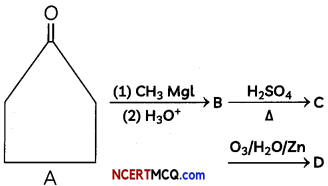OR
Give suitable chemical reactions for the following:
(A) Methanal gives methanol on catalytic hydrogenation in the presence of catalysts such as Ni, Pt or Pd.
(B) Methanal reacts with hydrogen cyanide to form methanal cyanohydrin.
(C) When methanal reacts with blue coloured Fehling’s solution, red precipitates of cuprous oxide (Cu20) are formed and the colour of Fehling’s solution changes from blue to red. (3)
Cyclopentanone reacts with methyl magnesium iodide (Grignard reagent) to form 1-methylcyclopentanoL The dehydration of 1-methylcyclopentanol with cone, sulphuric acid gives 1-methylcyclopent- 1-ene.

Reductive ozonolysis of 1-methylcyclopent-l- ene gives 5-oxohexanal.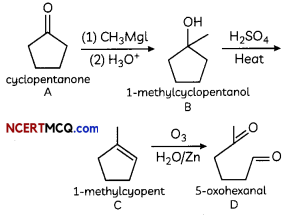OR
(A) Methanal gives methanol on catalytic hydrogenation in the presence of catalysts such as Ni, Pt or Pd.
Ni/Pt
HCHO → CH3OH

(B) Methanal reacts with hydrogen cyanide to form methanal cyanohydrin.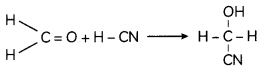(C) Methanal on reaction with Fehlings solution gives red precipitate and itself oxidises to sodium salt of methanoic acid.Question 11.
(A) Write the formula of an oxo-anion of chromium (Cr) in which it shows the oxidation state equal to its group number.
(B) Why do transition elements exhibit higher enthalpies-of atomization?
(C) Name the element showing the maximum number of oxidation states among the first series of transition metals from Sc (Z = 21) to Zn (Z = 30).
OR
The standard electrode potential for the M3+/M2+ half-cell gives the relative stability between M3+ and M2+. The reduction potential values are tabulated as below:
Reaction-Standard reduction potential (V)

 Reaction Standard reduction potential (V) Ti3+ + e– → Ti2+ -0.37 V3+ + e– → V2+ -0.26 Cr3+ + e– → Cr2+ -0.41 Mn3+ + e– → Cr2+ +1.51 Fe3+ + e– → Mn2+ +0.77 Co3+ + e– → Co2+ +1.81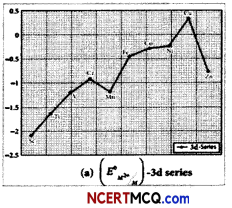(A) What does the negative values for titanium, vanadium and chromium indicate?
(B) What does reduction potentail of Mn3+/ Mn2+ indicate about the stability of these oxidation states?
(C) The E° value for the Mn3+/ Mn2+couple is much more positive than that for Cr3+/ Cr2+ couple or Fe3+/Fe2+ couple. (3)
(A) An oxo-anion of chromium (Cr) in which it shows the oxidation state equal to its group number is Cr2 O72-. Here, oxidation number of Cr is + 6 which is equal to its group number.

(B) The high enthalpies of atomization are due to a large number of unpaired electrons in their atoms. Therefore, they have stronger interatomic interactions and hence, stronger bonding between atoms. Thus, they have high enthalpies of atomization.

(C) Mn (Z=25) has the maximum number of unpaired electrons present in the d-subshell so it shows maximum oxidation states (+2 to +7)
OR
(A) The negative values for titanium, vanadium and chromium indicate that the higher oxidation state is preferred.

(B) The high reduction potential of Mn3+/ Mn2+ indicates Mn2+ is more stable than Mn3+ Mn3+ has a 3d4 configuration while that of Mn2+ is 3d5. The extra stability associated with a half filled d sub shell makes the reduction of Mn3+ very feasible (E° = + 1.51V).

(C) Because Mn3+ has the outer electronic configuration of 3d4 and Mn2+ has the outer electronic configuration of 3d5. Thus, the conversion of Mn3+ to Mn2+ will be a favourable reaction since 3d5 is a very stable configuration as it is half filled configuration. Hence, E° value for Mn3+/ Mn2+ couple is positive.
Fe3+ to Fe2+ undergoes a change in outer electronic configuration from 3d5 to 3d6. The configuration of the resultant are not stable

Related Theory:
Low reduction potential means there is a greater tendency for reduction to occur, while a lower one means there is a greater tendency for oxidation to occur.Section – C
(Section C-Question No 12 is case-based question carrying 5 marks.)

Question 12.
Amine, any member of a family of nitrogen- containing organic compounds that is derived, either in principle or in practice, from ammonia (NH3).
Naturally occurring amines include the alkaloids, which are present in certain plants; the catecholamine neurotransmitters (i.e., dopamine, epinephrine, and norepinephrine); and a local chemical mediator, histamine, that occurs in most animal tissues.
Most of the numerous methods for the preparation of amines may be broadly divided into two groups: (1) chemical reduction (replacement of oxygen with hydrogen atoms in the molecule) of members of several other classes of organic nitrogen compounds and (2) reactions of ammonia or amines with organic compounds.
Amines are classified as primary, secondary, or tertiary depending on whether one, two, or three of the hydrogen atoms of ammonia have been replaced by organic groups. In chemical notation these three classes are represented as RNH2, R2NH, and R3N, respectively. A fourth category consists of quaternary ammonium compounds, which are obtained by replacement of all four hydrogen atoms of the ammonium ion, NH4+; an anion is necessarily associated (R4N+X). Amines are also classified as aliphatic, having only aliphatic groups attached, or aromatic, having one or more aryl groups attached. They may be open-chain, in which the nitrogen is not part of a ring, or cyclic, in which it is a member of a ring (generally aliphatic). The carbylamine reaction, also known as Hofmann’s isocyanide test, is a chemical test for the detection of primary amines.
(A) Name two alkaloids present in neurotransmitters.
(B) Write the structure of n-methyl ethanamine.
(C) What are the two methods generally used for the preparation of amines?
(D) Write chemical equations for the following conversions: (5)
(i) Nitrobenzene to benzoic acid.
(ii) Benzyl chloride to 2-phenylethanamine
OR
Account for the following :
(i) Primary amines (R-NH2) have higher boiling point than tertiary amines (R3N).
(ii) Aniline does not undergo Friedel – Crafts reaction.
(A) Dopamine, Epinephrine

(B) H3C—H2C—NH—CH3

(C) (i) Chemical reduction (replacement of oxygen with hydrogen atoms in the molecule) of members of several other classes of organic nitrogen compounds

(ii) reactions of ammonia or amines with organic compounds.

(D) (i) Nirtobenzene to benzoic acid: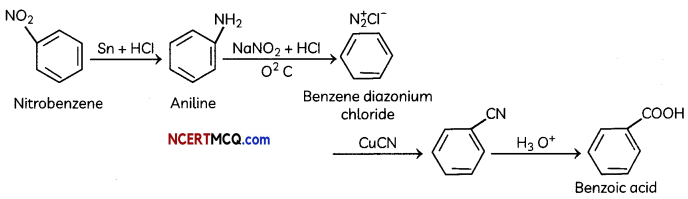(ii) Benzyl chloride to 2-phenylethanamineOR
(i) Due to the presence of two H-atoms on N-atom of primary amines, they undergo extensive intermolecular H-bonding while tertiary amines due to the absence of a H-atom on the N-atom, do not undergo H- bonding. As a result, primary amines have higher boiling points than 3° amines.

(ii) Aniline being a Lewis base reacts with Lewis acid AlCl3 to form a salt.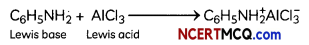As a result, N of aniline acquires positive charge and hence it acts as a strong deactivating group for electrophilic substitution reaction. Consequently, aniline does not undergo Friedel Craft reaction.